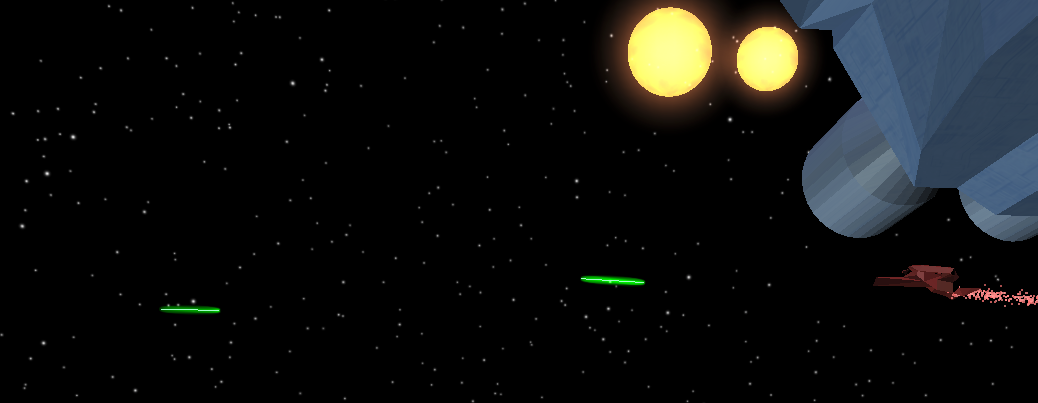For a recent school project, I set out to achieve a glow effect using three js for the stars and lasers in a space scene. I stumbled upon a very helpful blog post from Lee Stemkoski detailing his approach to a glow shader. With just a minor tweak, I was able to achieve the effects shown above and below. Interactive DemoThe glow effect works based on the idea that if you have a mesh with smooth, rounded corners the dot product of the view angle and the normal of a face will be highest at the center of the object, and lowest (approaching 0) at the edges. Using this property, we can effectively create a satisfying glow effect.

For each glowing object, we need the base geometry, and then another copy of that geometry at the same position, scaled up to be slightly larger. I used a scaling factor between 1 and 1.5. If the geometry is already smooth, then we can move on, otherwise use something like a subdivision modifier to smooth it out. In my case the sun was already a smooth sphere. For the lasers, I started with a cylinder and then smoothed it.

Then at every frame you need to update the view vector based on camera and object positions and that info to the shader.

``````let viewVector = new THREE.Vector3().subVectors( camera.position, object.glow.getWorldPosition());
object.glow.material.uniforms.viewVector.value = viewVector;
``````

The vertex shader calculates the dot product of the view vector and the normal, then raises it to a factor (6 in my case) that determines the relative intensity and sharpness of the glow. Higher powers are less intense since the dot product is always less than 1. For calculating the dot product the enhancement I made to the original shader is to calculate the `actual_normal` by multiplying the `modelMatrix` times the normal. That way, even if the geometry is rotated the dot product calculation will work as expected.

``````uniform vec3 viewVector;
varying float intensity;
void main() {
gl_Position = projectionMatrix * viewMatrix * modelMatrix * vec4( position, 1.0 );
vec3 actual_normal = vec3(modelMatrix * vec4(normal, 0.0));
intensity = pow( dot(normalize(viewVector), actual_normal), 6.0 );
}
``````

The fragment shader multiplies the color by the intensity you just calculated. That’s all it takes for this “good-enough” glow shader.

``````varying float intensity;
void main() {
vec3 glow = vec3(0, 1, 0) * intensity;
gl_FragColor = vec4( glow, 1.0 );
}
``````